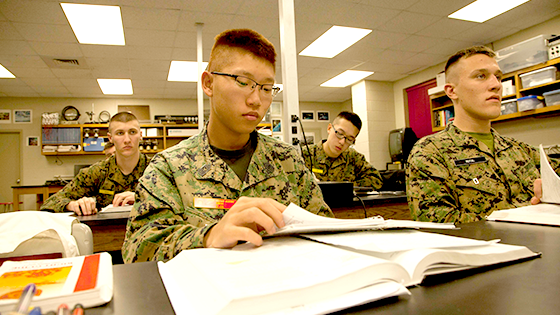# Course Catalog

Our curriculum is focuses on preparing students for college. Emphasis is placed on the core subjects.Math

The mathematics department focuses on helping all cadets develop the problem solving and higher order thinking skills necessary to ensure success in collegiate mathematics course work.

Our college preparatory sequence emphasizes a conceptual understanding of mathematics, which promotes flexible thinking and teaches cadets to apply the knowledge learned to other disciplines.

Fundamentals of Math

FOM is a transitional course in our math curriculum designed to automate the upper level skills of arithmetic while introducing abstract arithmetic concepts.

Pre-Algebra

Pre-Algebra is a middle school course that will guide one through the study of integers, one-step equations, inequalities and equations, graphs and functions, percent and probabilities. The Pre-Algebra objectives include: arithmetic properties, place value, arithmetic properties rounding whole numbers, arithmetic properties, regrouping whole numbers, factors and multiples, reading and interpreting data, measurement, fractions, decimals, negative numbers and coordinate plane, ratios, rates and proportions.

Algebra I

This course focuses on mathematical problem solving or the application of mathematical concepts in new situations. Cadets learn by working carefully designed problems that lead to the use of productive thought patterns utilizing concepts.

Algebra II

This course is designed to prepare cadets for pre-calculus. Cadets will continue to study area and volume and be introduced to more abstract geometric concepts, review prior algebra concepts and proceed through trigonometric ratios, rectangular and polar coordinates and on to changing from one coordinate system to the other.

Geometry

One of the main objectives of this course is to enhance cadets’ logical thinking skills. The course begins with an introduction to the basic concepts of geometry, such as lines, planes and points, then progresses to the study of various geometric shapes and their properties, and concludes with proofs.

Pre-College Algebra

The Pre-College Algebra class will allow students to review concepts from previous math courses. This course reviews Algebra I and Algebra II and presents students with Pre-Calculus concepts. This class is designed to prepare students for College Algebra.

Pre-Calculus

This course provides in-depth coverage of trigonometry, logarithms, analytic geometry and upper level algebraic concepts. Cadets expand their understanding of mathematical concepts through the use of symbolic reasoning and analytical methods to represent mathematical situations, to express generalizations and study the relationships between them.

With a teacher's recommendation, cadets accelerating in math may take the first year (pre-calculus) and then proceed to calculus. All other cadets will continue with advanced math. Pre-calculus and advanced math provide in-depth coverage of trigonometry, logarithms, analytic geometry and upper level algebraic concepts.

Calculus

An advanced placement (AP) course in calculus consists of a full high school academic year, which is comparable to college calculus coursework. It is expected that cadets who take AP calculus will seek college credit, college placement, or both, from institutions of higher learning.

Statistics

This course introduces cadets to the major concepts and tools for collecting, analyzing and drawing conclusions from data. Cadets will be exposed to four major concepts: exploring data, planning a study, probability as it relates to distribution of data and inferential reasoning and modeling.

Special Topics in Math

This course is designed for those who are about to graduate and who lack skills and basic concepts in math to succeed in higher math courses. In this class we identify the areas of each student’s weaknesses and help him better understand those areas, from basic operations to solving complex equations, graphing and solving systems of equations and higher.